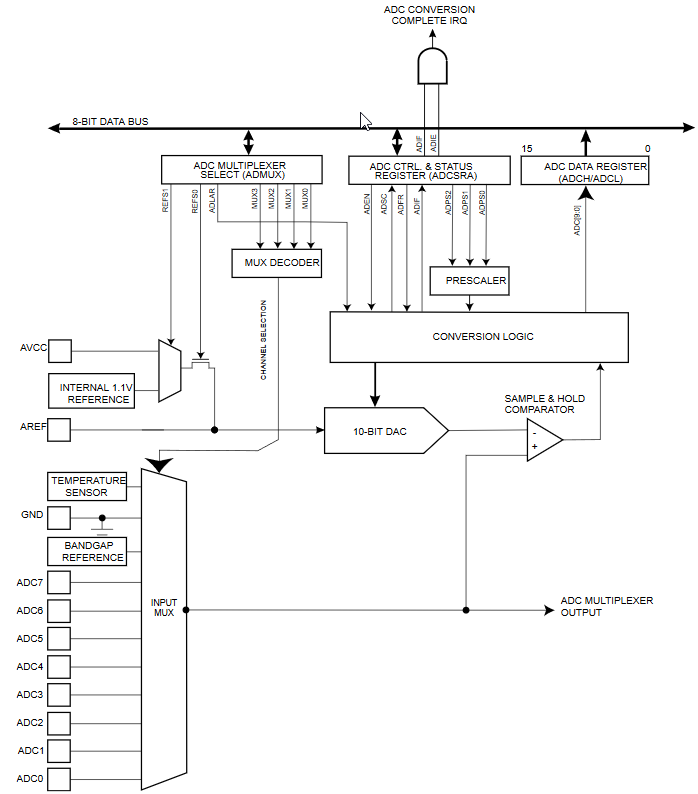# A/D converter - an eight bit resolution

Zápisník experimentátora

Hierarchy:

The A/D converter in Arduino Uno has a resolution of 10 bits. If you do not need such a large resolution, we can also use a resolution of 8 bits. This will allow us to convert the analog value to a digital value that has exactly 8 bits. This is done by adjusting bit alignment in the resulting 16-bit register. Using an 8 bit A / D conversion has the advantage that we do not have to comply with the recommended 50 kHz - 200 kHz conversion frequency and we can also use higher frequencies.## Example

The example is almost the same as the previous examples (A/D converter without blocking). It varies only in the `ADMUX.ADLAR` bit setting that adjusts the alignment of the result. With this setting, just read the contents of the `ADCH` registry to get the resulting 8 bit result.

``````const byte adcPin = A0; // = 14 (pins_arduino.h)

void setup() {
Serial.begin(115200);

| ((adcPin - 14) & 0x07); // Arduino Uno to ADC pin
}

void loop() {
}

// The ADC clears the bit when done
Serial.println(value);
delay(500);
}
}``````

## Source code

The source code is located on the GitHub server.

03.11.2018